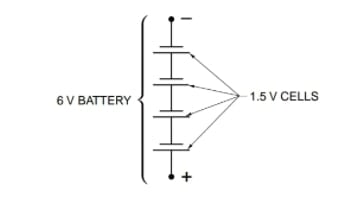Technical Article

# Choosing the Right Battery Configuration

September 26, 2021 by Alex Roderick

## Today, portable electronics are everywhere, and batteries are used to power these devices. Batteries are an example of how electrical energy is generated using a chemical reaction.

Batteries have two or more cells. A cell is a device that produces electricity at a fixed voltage with limited current capacity. According to this definition, the small batteries sold to consumers are not batteries but cells. The different cell types have different voltages and current capacities and support different loads (see Figure 1).

 Cell Type Voltage (V) Capacity (mAh) Typical Load (mA) D 1.5 13,000 200 C 1.5 6000 100 AA 1.5 2400 50 AAA 1.5 1000 10 9 V 9 500 15
##### Figure 1. Different battery cell types have different voltages and current capacities and support different loads.

The different capacities indicate how long a cell will last for a given load and is given in amp-hours (Ah). For example, if an AA cell was used with a 10 mA load, then the cell would last 240 hours (2400 mAh ÷ 10 mA = 240 hours). The typical load ensures maximum cell life. Higher loads reduce cell life below the listed capacity.

## An Example Problem

A portable voltage source (battery) must be configured to support an application that requires 6.0 V with a load of 600 mA. The application also requires battery life greater than 100 hr (see Figure 2).## The Solution

To start, select the cell configuration that provides the proper voltage. The cells being used provide 1.5 V each. To increase the voltage, a number of cells are connected in series. To meet the required voltage, the number of required series cells must be determined.

${{V}_{T}}=number\text{ }of\text{ }cells\text{ }(N)\times 1.5\text{ }V$

$6\text{ }V=N\times 1.5\text{ }V$

$N=\frac{6V}{1.5V}=4$

To create the required voltage source, a total of four 1.5 V cells are connected in series to form a 6 V battery. With the voltage sources connected in series, the current capability is limited to that provided by an individual cell. For example, if four AA cells are used, the 6 V battery has a capacity of 2400 mAh, supporting a typical load up to 50 mA. The required battery capacity is set by the current load and time. This is found by multiplying the current load and hours together for amp-hours.

Capacity needed (in mAh) = current load (in mA) × time (in hours)

Capacity needed (in mAh) = 600 mA × 100 h

Capacity needed (in mAh) = 60,000 mAh

Since a single cell type cannot supply this current capacity, the capacity must be increased. To increase the battery current capacity, parallel batteries are added. Each parallel battery must match the original battery voltage. In this application, each parallel battery must be 6 V. To find the number of parallel batteries required (for a size D cell battery), the required capacity is divided by the battery capacity as follows:

$N=\frac{{{C}_{T}}}{{{C}_{B}}}$

Where

$$N$$ = number of parallel batteries

$$CT$$ = total capacity required (in mAh)

$$CB$$ = battery capacity (in mAh)

For this application:

N = 60,000 mAh ÷ 13,000 mAh

N = 4.62

N = 5 parallel batteries

Finally, maximum battery life must be verified by ensuring that the typical load is not exceeded. For a D cell battery, the typical load is 200 mA. Each parallel battery supports the typical load. To find the supported typical load, multiply the number of parallel batteries by the typical cell load.

TLS = TCL × N

where

TLS = typical load support (in mA)

TCL = typical cell load (in mA)

N = number of parallel batteries

For this application:

TLS = 200 mA × 5

TLS = 1000 mA

 6 V Battery – 65,000 mAh Capacity And 1000 mA Typical Load##### Figure 3. A total of 20 D cells is required for the battery configuration.

The battery configuration in this application supports a typical load of 1000 mA, which is greater than the 600 mA requirement. As shown in Figure 3, the final battery configuration required for this application consists of a total of 20 D cells (five parallel batteries with each branch containing four D cells connected in series).

Featured Image used courtesy of Penny Electric